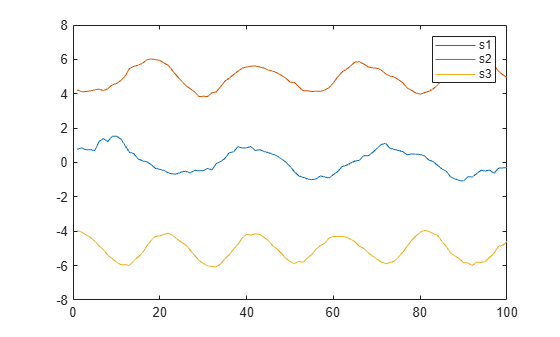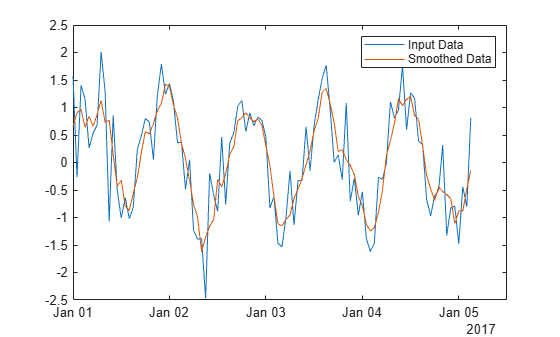# smoothdata

Smooth noisy data

## Syntax

``B = smoothdata(A)``
``B = smoothdata(A,dim)``
``B = smoothdata(___,method)``
``B = smoothdata(___,method,window)``
``B = smoothdata(___,nanflag)``
``B = smoothdata(___,Name,Value)``
``````[B,window] = smoothdata(___)``````

## Description

example

````B = smoothdata(A)` returns a moving average of the elements of a vector using a fixed window length that is determined heuristically. The window slides down the length of the vector, computing an average over the elements within each window. If `A` is a matrix, then `smoothdata` computes the moving average down each column.If `A` is a multidimensional array, then `smoothdata` operates along the first dimension whose size does not equal 1.If `A` is a table or timetable with numeric variables, then `smoothdata` operates on each variable separately. ```

example

````B = smoothdata(A,dim)` operates along the dimension `dim` of `A`. For example, if `A` is a matrix, then `smoothdata(A,2)` smooths the data in each row of `A`.```

example

````B = smoothdata(___,method)` specifies the smoothing method for either of the previous syntaxes. For example, ```B = smoothdata(A,'sgolay')``` uses a Savitzky-Golay filter to smooth the data in `A`.```

example

````B = smoothdata(___,method,window)` specifies the length of the window used by the smoothing method. For example, `smoothdata(A,'movmedian',5)` smooths the data in `A` by taking the median over a five-element sliding window.```

example

````B = smoothdata(___,nanflag)` specifies how `NaN` values are treated for any of the previous syntaxes. `'omitnan'` ignores `NaN` values and `'includenan'` includes them when computing within each window.```

example

````B = smoothdata(___,Name,Value)` specifies additional parameters for smoothing using one or more name-value pair arguments. For example, if `t` is a vector of time values, then `smoothdata(A,'SamplePoints',t)` smooths the data in `A` relative to the times in `t`.```

example

``````[B,window] = smoothdata(___)``` also returns the moving window length.```

## Examples

collapse all

Create a vector containing noisy data, and smooth the data with a moving average. Plot the original and smoothed data.

```x = 1:100; A = cos(2*pi*0.05*x+2*pi*rand) + 0.5*randn(1,100); B = smoothdata(A); plot(x,A,'-o',x,B,'-x') legend('Original Data','Smoothed Data')```Create a matrix whose rows represent three noisy signals. Smooth the three signals using a moving average, and plot the smoothed data.

```x = 1:100; s1 = cos(2*pi*0.03*x+2*pi*rand) + 0.5*randn(1,100); s2 = cos(2*pi*0.04*x+2*pi*rand) + 0.4*randn(1,100) + 5; s3 = cos(2*pi*0.05*x+2*pi*rand) + 0.3*randn(1,100) - 5; A = [s1; s2; s3]; B = smoothdata(A,2); plot(x,B(1,:),x,B(2,:),x,B(3,:))```Smooth a vector of noisy data with a Gaussian-weighted moving average filter. Display the window length used by the filter.

```x = 1:100; A = cos(2*pi*0.05*x+2*pi*rand) + 0.5*randn(1,100); [B, window] = smoothdata(A,'gaussian'); window```
```window = 4 ```

Smooth the original data with a larger window of length 20. Plot the smoothed data for both window lengths.

```C = smoothdata(A,'gaussian',20); plot(x,B,'-o',x,C,'-x') legend('Small Window','Large Window')```Create a noisy vector containing `NaN` values, and smooth the data ignoring `NaN`, which is the default.

```A = [NaN randn(1,48) NaN randn(1,49) NaN]; B = smoothdata(A);```

Smooth the data including `NaN` values. The average in a window containing `NaN` is `NaN`.

`C = smoothdata(A,'includenan');`

Plot the smoothed data in `B` and `C`.

```plot(1:100,B,'-o',1:100,C,'-x') legend('Ignore NaN','Include NaN')```Create a vector of noisy data that corresponds to a time vector `t`. Smooth the data relative to the times in `t`, and plot the original data and the smoothed data.

```x = 1:100; A = cos(2*pi*0.05*x+2*pi*rand) + 0.5*randn(1,100); t = datetime(2017,1,1,0,0,0) + hours(0:99); B = smoothdata(A,'SamplePoints',t); plot(t,A,'-o',t,B,'-x') legend('Original Data','Smoothed Data')```## Input Arguments

collapse all

Input array, specified as a vector, matrix, multidimensional array, table, or timetable. If `A` is a table or timetable, then either the variables must be numeric, or you must use the `'DataVariables'` name-value pair to list numeric variables explicitly. Specifying variables is useful when you are working with a table that also contains non-numeric variables.

Data Types: `double` | `single` | `int8` | `int16` | `int32` | `int64` | `uint8` | `uint16` | `uint32` | `uint64` | `logical` | `table` | `timetable`

Complex Number Support: Yes

Dimension to operate along, specified as a positive integer scalar. If no value is specified, then the default is the first array dimension whose size does not equal 1.

Consider a matrix `A`.

`B = smoothdata(A,1)` smooths the data in each column of `A`.`B = smoothdata(A,2)` smooths the data in each row of `A`.When `A` is a table or timetable, `dim` is not supported. `smoothdata` operates along each table or timetable variable separately.

Data Types: `double` | `single` | `int8` | `int16` | `int32` | `int64` | `uint8` | `uint16` | `uint32` | `uint64`

Smoothing method, specified as one of the following:

• `'movmean'` — Moving average over each window of `A`. This method is useful for reducing periodic trends in data.

• `'movmedian'` — Moving median over each window of `A`. This method is useful for reducing periodic trends in data when outliers are present.

• `'gaussian'` — Gaussian-weighted moving average over each window of `A`.

• `'lowess'` — Linear regression over each window of `A`. This method can be computationally expensive, but results in fewer discontinuities.

• `'loess'` — Quadratic regression over each window of `A`. This method is slightly more computationally expensive than `'lowess'`.

• `'rlowess'` — Robust linear regression over each window of `A`. This method is a more computationally expensive version of the method `'lowess'`, but it is more robust to outliers.

• `'rloess'` — Robust quadratic regression over each window of `A`. This method is a more computationally expensive version of the method `'loess'`, but it is more robust to outliers.

• `'sgolay'` — Savitzky-Golay filter, which smooths according to a quadratic polynomial that is fitted over each window of `A`. This method can be more effective than other methods when the data varies rapidly.

Window length, specified as a positive integer scalar, a two-element vector of positive integers, a positive duration scalar, or a two-element vector of positive durations.

When `window` is a positive integer scalar, then the window is centered about the current element and contains `window-1` neighboring elements. If `window` is even, then the window is centered about the current and previous elements. If `window` is a two-element vector of positive integers `[b f]`, then the window contains the current element, `b` elements backward, and `f` elements forward.

When `A` is a timetable or when `'SamplePoints'` is specified as a `datetime` or `duration` vector, `window` must be of type `duration`, and the window is computed relative to the sample points.

When the window length is also specified as an output argument, the output value matches the input value.

Data Types: `double` | `single` | `int8` | `int16` | `int32` | `int64` | `uint8` | `uint16` | `uint32` | `uint64` | `duration`

`NaN` condition, specified as one of the following values:

• `'omitnan'` — Ignore `NaN` values in the input. If a window contains all `NaN` values, then `smoothdata` returns `NaN`.

• `'includenan'` — Include `NaN` values when computing within each window, resulting in `NaN`.

### Name-Value Pair Arguments

Specify optional comma-separated pairs of `Name,Value` arguments. `Name` is the argument name and `Value` is the corresponding value. `Name` must appear inside quotes. You can specify several name and value pair arguments in any order as `Name1,Value1,...,NameN,ValueN`.

Example: `smoothdata(A,'SmoothingFactor',0.5)`
Data Options

collapse all

Sample points, specified as the comma-separated pair consisting of `'SamplePoints'` and a vector. The sample points represent the x-axis locations of the data in `A`, and must be sorted and contain unique values. Sample points do not need to be uniformly sampled. The default sample points vector is `[1 2 3 ...]`.

Note

This name-value pair is not supported when the input data is a `timetable`. Timetables always use the vector of row times as the sample points. To use different sample points, you must edit the timetable so that the row times contain the desired sample points.

Moving windows are defined relative to the sample points. For example, if `t` is a vector of times corresponding to the input data, then `smoothdata(rand(1,10),3,'SamplePoints',t)` has a window that represents the time interval between `t(i)-1.5` and `t(i)+1.5`.

When the sample points vector has data type `datetime` or `duration`, then the moving window length must have type `duration`.

Example: `smoothdata(T,'SamplePoints',0:0.1:10)`

Data Types: `double` | `single` | `int8` | `int16` | `int32` | `int64` | `uint8` | `uint16` | `uint32` | `uint64` | `datetime` | `duration`

Table variables to operate on, specified as the comma-separated pair consisting of `'DataVariables'` and one of the options in this table. The `'DataVariables'` value indicates which variables of the input table to smooth. Other variables in the table not specified by `'DataVariables'` pass through to the output without being operated on.

OptionDescriptionExamples
Variable name

A character vector or scalar string specifying a single table variable name

`'Var1'`

`"Var1"`

Vector of variable names

A cell array of character vectors or string array where each element is a table variable name

`{'Var1' 'Var2'}`

`["Var1" "Var2"]`

Scalar or vector of variable indices

A scalar or vector of table variable indices

`1`

`[1 3 5]`

Logical vector

A logical vector whose elements each correspond to a table variable, where `true` includes the corresponding variable and `false` excludes it

`[true false true]`

Function handle

A function handle that takes a table variable as input and returns a logical scalar

`@isnumeric`

`vartype` subscript

A table subscript generated by the `vartype` function

`vartype('numeric')`

Example: ```smoothdata(T,'DataVariables',["Var1" "Var2" "Var4"])```

Smoothing Options

collapse all

Window size factor, specified as the comma-separated pair consisting of `'SmoothingFactor'` and a scalar ranging from 0 to 1. Generally, the value of `'SmoothingFactor'` adjusts the level of smoothing by scaling the heuristic window size. Values near 0 produce smaller moving window lengths, resulting in less smoothing. Values near 1 produce larger moving window lengths, resulting in more smoothing. In some cases, depending on the input data from which the heuristic window size is determined, the value of `'SmoothingFactor'` may not have a significant impact on the window size used by `smoothdata`.

`'SmoothingFactor'` is 0.25 by default and can only be specified when `window` is not specified.

Data Types: `double` | `single` | `int8` | `int16` | `int32` | `int64` | `uint8` | `uint16` | `uint32` | `uint64`

Savitzky-Golay degree, specified as the comma-separated pair consisting of `'Degree'` and a nonnegative integer. This name-value pair can only be specified when `'sgolay'` is the specified smoothing method. The value of `'Degree'` corresponds to the degree of the polynomial in the Savitzky-Golay filter that fits the data within each window, which is 2 by default.

The value of `'Degree'` must be less than the window length for uniform sample points. For nonuniform sample points, the value must be less than the maximum number of points in any window.

## Output Arguments

collapse all

Output array, returned as a vector, matrix, multidimensional array, table, or timetable. `B` is the same size as `A`.

Window length, returned as a positive integer scalar, a two-element vector of positive integers, a positive duration scalar, or a two-element vector of positive durations.

When `window` is specified as an input argument, the output value matches the input value. When `window` is not specified as an input argument, then its value is the scalar heuristically determined by `smoothdata` based on the input data.

Data Types: `double` | `single` | `int8` | `int16` | `int32` | `int64` | `uint8` | `uint16` | `uint32` | `uint64` | `duration`

## Algorithms

When the window size for the smoothing method is not specified, `smoothdata` computes a default window size based on a heuristic. For a smoothing factor τ, the heuristic estimates a moving average window size that attenuates approximately 100*τ percent of the energy of the input data.